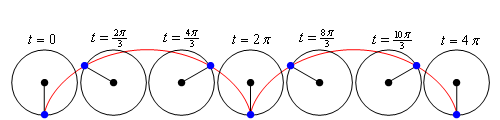Paul's Online Notes
Home / Calculus II / Parametric Equations and Polar Coordinates / Area with Parametric Equations
Show Mobile Notice Show All Notes Hide All Notes
Mobile Notice
You appear to be on a device with a "narrow" screen width (i.e. you are probably on a mobile phone). Due to the nature of the mathematics on this site it is best views in landscape mode. If your device is not in landscape mode many of the equations will run off the side of your device (should be able to scroll to see them) and some of the menu items will be cut off due to the narrow screen width.

### Section 9.3 : Area with Parametric Equations

In this section we will find a formula for determining the area under a parametric curve given by the parametric equations,

$x = f\left( t \right)\hspace{0.25in}\hspace{0.25in}y = g\left( t \right)$

We will also need to further add in the assumption that the curve is traced out exactly once as $$t$$ increases from $$\alpha$$ to $$\beta$$.

We will do this in much the same way that we found the first derivative in the previous section. We will first recall how to find the area under $$y = F\left( x \right)$$ on $$a \le x \le b$$.

$A = \int_{{\,a}}^{{\,b}}{{F\left( x \right)\,dx}}$

We will now think of the parametric equation $$x = f\left( t \right)$$ as a substitution in the integral. We will also assume that $$a = f\left( \alpha \right)$$ and $$b = f\left( \beta \right)$$ for the purposes of this formula. There is actually no reason to assume that this will always be the case and so we’ll give a corresponding formula later if it’s the opposite case ($$b = f\left( \alpha \right)$$ and $$a = f\left( \beta \right)$$).

So, if this is going to be a substitution we’ll need,

$dx = f'\left( t \right)\,dt$

Plugging this into the area formula above and making sure to change the limits to their corresponding $$t$$ values gives us,

$A = \int_{{\,\alpha }}^{{\,\beta }}{{F\left( {f\left( t \right)} \right)\,f'\left( t \right)dt}}$

Since we don’t know what $$F\left( x \right)$$ is we’ll use the fact that

$y = F\left( x \right) = F\left( {f\left( t \right)} \right) = g\left( t \right)$

and we arrive at the formula that we want.

#### Area Under Parametric Curve, Formula I

$A = \int_{{\,\alpha }}^{{\,\beta }}{{g\left( t \right)\,f'\left( t \right)dt}}$

Now, if we should happen to have $$b = f\left( \alpha \right)$$ and $$a = f\left( \beta \right)$$ the formula would be,

#### Area Under Parametric Curve, Formula II

$A = \int_{{\,\beta }}^{{\,\alpha }}{{g\left( t \right)\,f'\left( t \right)dt}}$

Let’s work an example.

Example 1 Determine the area under the parametric curve given by the following parametric equations.

$x = 6\left( {\theta - \sin \theta } \right)\hspace{0.5in}y = 6\left( {1 - \cos \theta } \right)\hspace{0.5in}0 \le \theta \le 2\pi$
Show Solution

First, notice that we’ve switched the parameter to $$\theta$$ for this problem. This is to make sure that we don’t get too locked into always having $$t$$ as the parameter.

Now, we could graph this to verify that the curve is traced out exactly once for the given range if we wanted to. We are going to be looking at this curve in more detail after this example so we won’t sketch its graph here.

There really isn’t too much to this example other than plugging the parametric equations into the formula. We’ll first need the derivative of the parametric equation for $$x$$ however.

$\frac{{dx}}{{d\theta }} = 6\left( {1 - \cos \theta } \right)$

The area is then,

\begin{align*}A & = \int_{{\,0}}^{{\,2\pi }}{{36{{\left( {1 - \cos \theta } \right)}^2}\,d\theta }}\\ & = 36\int_{{\,0}}^{{\,2\pi }}{{1 - 2\cos \theta + {{\cos }^2}\theta \,d\theta }}\\ & = 36\int_{{\,0}}^{{\,2\pi }}{{\frac{3}{2} - 2\cos \theta + \frac{1}{2}\cos \left( {2\theta } \right)\,d\theta }}\\ & = 36\left. {\left( {\frac{3}{2}\theta - 2\sin \theta + \frac{1}{4}\sin \left( {2\theta } \right)} \right)} \right|_0^{2\pi }\\ & = 108\pi \end{align*}

The parametric curve (without the limits) we used in the previous example is called a cycloid. In its general form the cycloid is,

$x = r\left( {\theta - \sin \theta } \right)\hspace{0.25in}\hspace{0.25in}y = r\left( {1 - \cos \theta } \right)$

The cycloid represents the following situation. Consider a wheel of radius $$r$$. Let the point where the wheel touches the ground initially be called $$P$$. Then start rolling the wheel to the right. As the wheel rolls to the right trace out the path of the point $$P$$. The path that the point $$P$$ traces out is called a cycloid and is given by the equations above. In these equations we can think of $$\theta$$ as the angle through which the point $$P$$ has rotated.

Here is a cycloid sketched out with the wheel shown at various places. The blue dot is the point $$P$$ on the wheel that we’re using to trace out the curve.From this sketch we can see that one arch of the cycloid is traced out in the range $$0 \le \theta \le 2\pi$$. This makes sense when you consider that the point $$P$$ will be back on the ground after it has rotated through an angle of $$2\pi$$.